The LOGISTIC Procedure
 Score Statistics and Tests

To understand the general form of the score statistics, letbe the vector of first partial derivatives of the log likelihood with respect to the parameter vector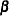, and let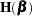be the matrix of second partial derivatives of the log likelihood with respect to. That is,is the gradient vector, andis the Hessian matrix. Let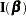be eitheror the expected value of. Consider a null hypothesis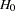. Letbe the MLE ofunder. The chi-square score statistic for testingis defined by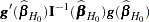and it has an asymptotic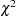distribution withdegrees of freedom under, whereis the number of restrictions imposed onby.

### Residual Chi-Square

When you use SELECTION=FORWARD, BACKWARD, or STEPWISE, the procedure calculates a residual chi-square score statistic and reports the statistic, its degrees of freedom, and the p-value. This section describes how the statistic is calculated.

Suppose there areexplanatory effects of interest. The full cumulative response model has a parameter vectorwhere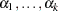are intercept parameters, and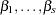are the common slope parameters for theexplanatory effects. The full generalized logit model has a parameter vector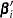where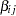is the slope parameter for theth effect in theth logit.

Consider the null hypothesis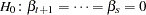, where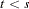for the cumulative response model, and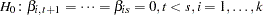, for the generalized logit model. For the reduced model withexplanatory effects, letbe the MLEs of the unknown intercept parameters, let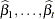be the MLEs of the unknown slope parameters, and let, be those for the generalized logit model. The residual chi-square is the chi-square score statistic testing the null hypothesis; that is, the residual chi-square iswhere for the cumulative response model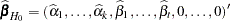, and for the generalized logit model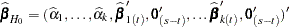, where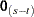denotes a vector of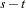zeros.

The residual chi-square has an asymptotic chi-square distribution withdegrees of freedom (for the generalized logit model). A special case is the global score chi-square, where the reduced model consists of theintercepts and no explanatory effects. The global score statistic is displayed in the "Testing Global Null Hypothesis: BETA=0" table. The table is not produced when the NOFIT option is used, but the global score statistic is displayed.

### Testing Individual Effects Not in the Model

These tests are performed when you specify SELECTION=FORWARD or STEPWISE, and are displayed when the DETAILS option is specified. In the displayed output, the tests are labeled "Score Chi-Square" in the "Analysis of Effects Not in the Model" table and in the "Summary of Stepwise (Forward) Selection" table. This section describes how the tests are calculated.

Suppose thatintercepts andexplanatory variables (say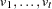) have been fit to a model and thatis another explanatory variable of interest. Consider a full model with theintercepts and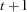explanatory variables (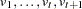) and a reduced model withexcluded. The significance ofadjusted forcan be determined by comparing the corresponding residual chi-square with a chi-square distribution with one degree of freedom (degrees of freedom for the generalized logit model).

### Testing the Parallel Lines Assumption

For an ordinal response, PROC LOGISTIC performs a test of the parallel lines assumption. In the displayed output, this test is labeled "Score Test for the Equal Slopes Assumption" when the LINK= option is NORMIT or CLOGLOG. When LINK=LOGIT, the test is labeled as "Score Test for the Proportional Odds Assumption" in the output. For small sample sizes, this test might be too liberal (Stokes, Davis, and Koch; 2000). This section describes the methods used to calculate the test.

For this test the number of response levels,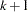, is assumed to be strictly greater than 2. Letbe the response variable taking values. Suppose there areexplanatory variables. Consider the general cumulative model without making the parallel lines assumptionwhere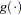is the link function, andis a vector of unknown parameters consisting of an intercept
andslope parameters. The parameter vector for this general cumulative model is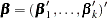Under the null hypothesis of parallelism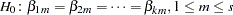, there is a single common slope parameter for each of theexplanatory variables. Let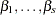be the common slope parameters. Letandbe the MLEs of the intercept parameters and the common slope parameters. Then, under, the MLE ofis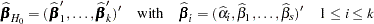and the chi-square score statistic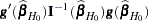has an asymptotic chi-square distribution withdegrees of freedom. This tests the parallel lines assumption by testing the equality of separate slope parameters simultaneously for all explanatory variables.Previous Page | Next Page | Top of Page